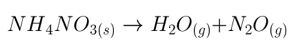# MCAT Chemistry Question — Nitrous Oxide

Amateur chemists make nitrous oxide at home using this (unbalanced) reaction:How many grams of NH4NO3 are required to produce 134.4 L of mixed gas if products are at STP?

(Do NOT heat the ammonium nitrate above 240°C or it may detonate)

1. 160
2. 240
3. 960
4. 1440
##### Click for Explanation

This solution has 3 steps: balance the equation, determine the required number of moles of product and reactant, and calculate the mass of reactant necessary. The reaction as written is unbalanced. In the balanced equation, 2 moles of H2O and 1 mole of N2O are made for each 1 mole of NH4NO3. 3 moles of product gas result from each mole of reactant. 22.4 L of gas corresponds to 1 mole of gas at STP, and the specified 134.4 L indicates 6 moles of product gases. 6 moles of mixed product gas requires 2 moles of reactant. NH4NO3 weighs 80 g/mole, so 160 g are required.

A. 160, correct. 2 moles of NH4NO3 yields 4 moles of H2O and 2 moles of N2O, for 6 moles of mixed product gas occupying 134.4 L at STP.

B. 240, incorrect, This answer results from using the unbalanced equation as written.

C. 960, incorrect, This answer results from using the unbalanced equation and calculating the mass of 12 moles NH4NO3 for 6 moles N2O product rather than mixed gas.

D. 1440, incorrect, This answer results from using the correct balanced equation and calculating the mass of 18 moles of NH4NO3 for 6 moles of N2O product rather than mixed gas.

## Want more MCAT practice?

### We’ve got options for every schedule and learning style!

From the best online MCAT course created by top instructors with 524+ MCAT scores to the most representative full-length practice exams and private tutoring, we can custom tailor your MCAT prep to your goals!

Not sure which option is right for you? Schedule a free MCAT consultation with an MCAT expert using the form below. No obligation, just expert advice.

Create your Free Account to access our MCAT Flashcards